Two equal bar magnets are kept as shown in the figure. The direction of resultant magnetic field, indicated by arrow head at the point P is (approximately)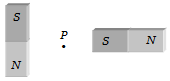1.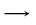2.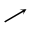3.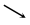4.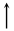Concept Questions :-

Bar magnet
High Yielding Test Series + Question Bank - NEET 2020

Difficulty Level:

A straight wire carrying a current i is turned into a circular loop. If the magnitude of the magnetic moment associated with it in M.K.S. unit is M, the length of wire will be

1. $4\mathrm{\pi iM}$                          2. $\sqrt{\frac{4\mathrm{\pi M}}{i}}$

3. $\sqrt{\frac{4\mathrm{\pi i}}{M}}$                        4. $\frac{M\mathrm{\pi }}{4i}$

Concept Questions :-

Magnetic moment
High Yielding Test Series + Question Bank - NEET 2020

Difficulty Level:

Two similar bar magnets P and Q, each of magnetic moment M, are taken. If P is cut along its axial line and Q is cut along its equatorial line, all the four pieces obtained have

1. Equal pole strength          2. Magnetic moment M/4

3. Magnetic moment M/2      4. Magnetic moment M

Concept Questions :-

Bar magnet
High Yielding Test Series + Question Bank - NEET 2020

Difficulty Level:

A magnet of magnetic moment  is placed along the x-axis in a magnetic field $\stackrel{\to }{B}=\left(0.5\stackrel{^}{i}+3.0\stackrel{^}{j}\right)T$. The torque acting on the magnet is

1. 175 $\stackrel{^}{k}$ N-m

2. 150 $\stackrel{^}{k}$ N-m

3. 75 $\stackrel{^}{k}$ N-m

4. 25$\sqrt{37}$ $\stackrel{^}{k}$ N-m

Concept Questions :-

Analogy between electrostatics and magnetostatics
High Yielding Test Series + Question Bank - NEET 2020

Difficulty Level:

Two lines of force due to a bar magnet

1. Intersect at the neutral point

2. Intersect near the poles of the magnet

3. Intersect on the equatorial axis of the magnet

4. Do not intersect at all

Concept Questions :-

Magnetic field and field lines
High Yielding Test Series + Question Bank - NEET 2020

Difficulty Level:

The magnetic field lines due to a bar magnet are correctly shown in

1.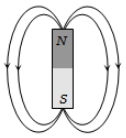2.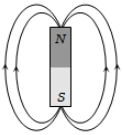3.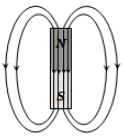4.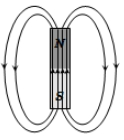Concept Questions :-

Magnetic field and field lines
High Yielding Test Series + Question Bank - NEET 2020

Difficulty Level:

A bar magnet of length 3 cm has points A and B along its axis at distances of 24 cm and 48 cm on the opposite sides. Ratio of magnetic fields at these points will be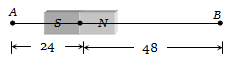(a) 8                               (b)$\frac{1}{2\sqrt{2}}$

(c) 3                               (d) 4

Concept Questions :-

Earth's magnetism
High Yielding Test Series + Question Bank - NEET 2020

Difficulty Level:

A dip needle in a plane perpendicular to magnetic meridian will remain

(a) Vertical

(b) Horizontal

(c) In any direction

(d) At an angle of dip to the horizontal

Concept Questions :-

Earth's magnetism
High Yielding Test Series + Question Bank - NEET 2020

Difficulty Level:

If the angles of dip at two places are 30o and 45o respectively, then the ratio of horizontal  components of earth's magnetic field at the two places will be

(a) $\sqrt{3}:\sqrt{2}$                       (b) $1:\sqrt{2}$

(c) $1:\sqrt{3}$                          (d) 1:2

Concept Questions :-

Earth's magnetism
High Yielding Test Series + Question Bank - NEET 2020

Difficulty Level:

A line passing through places having zero value of magnetic dip is called

(a) Isoclinic line             (b) Agonic line

(c) Isogonic line             (d) Aclinic line

Concept Questions :-

Earth's magnetism